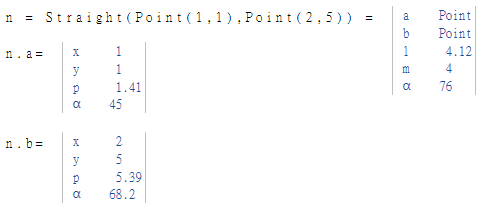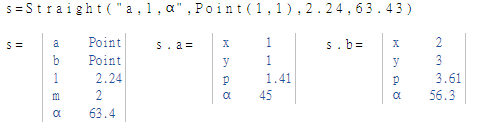# Class Straight

Calculation of the properties of a straight line

## Description

The class Straight calculates properties of straight lines between 2 points.

The the points $$a$$ and $$b$$ are default arguments. That means the points can be passed as an argument without a command string (see example below).

## Properties

The following properties can be queried as a result or passed as an argument.

#### Options

a Start Point default
b End Point defaut
l Length of the line
m Gradient is the steepness of the line
alpha α Angle to the X-axis

## Syntax

Object = Straight (Point a, Point b)

Object = Straight ("arg names", arguments)

Value = Straight ("get -> arg names", arguments)

## ExamplesThe next example shows how to calculate the end position of a line when the length and angle to the x-axis are known. Starting position $$a$$ is assumed to be $$x = 1$$ and $$y = 1$$.